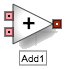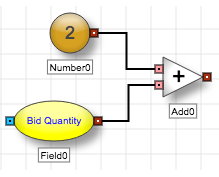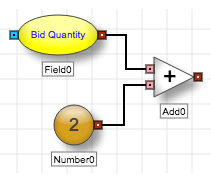# Arithmetic blocks

Arithmetic blocksThe Add block adds the first input number and the second input number and outputs the sum.

Example The Add block adds 2 to the bid quantity.## More about Flip for Sell Order

When the Flip for Sell Order option is enabled, the Add block will either perform an addition or a subtraction operation, depending on the value of the Order Side Variable (see: Flip For Sell Order Functionality for more information).

Note If you enable Flip for Sell Order, the order of the inputs matter because it subtracts the two inputs when flipped.

As an illustration, the previous example might produce undesired results if you enable Flip for Sell Order. In the Add block, the first input is 2 and the second input is the bid quantity. If flipped, the Add block would subtract the bid quantity from 2, which is probably not the intended behavior. If you plan to enable Flip for Sell Order, you likely want to configure the Add block as follows.

Example Arrange inputs to work when flipped.## Block properties

To customize the block, update the Block Properties.

Property Description
Name Name to display beneath the block on the ADL canvas
Flip for Sell Order

Whether to enable a single algorithm to act either as buy or sell side routine as needed

See Flip For Sell Order Functionality for more information.